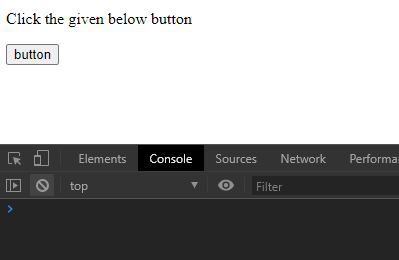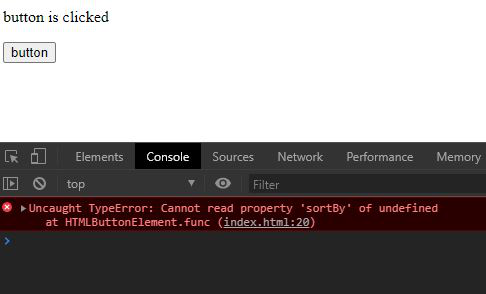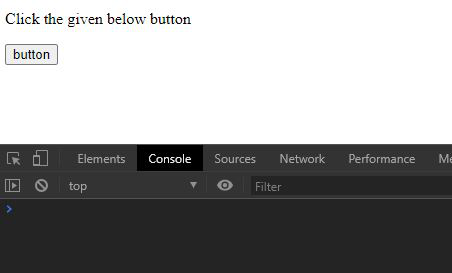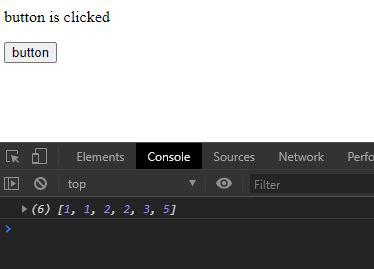# Underscore.js _.noConflict() Function

• Last Updated : 28 Oct, 2021

Underscore.js is a library in javascript that makes operations on arrays, string, objects much easier and handy.
The _.noConflict() function is used to create a reference of the global underscore object “_” to another variable.

Note: It is very necessary to link the underscore CDN before going and using underscore functions in the browser. When linking the underscore.js CDN The “_” is attached to the browser as a global variable.

Syntax:

`_.noConflict()`

Parameters: This function does not accept any parameter.

Return Value: It returns the reference to the global underscore variable.

Example 1: When noConflict() function is not used and using “underscore” variable.

## HTML

 `` `<``html``>`   `<``head``>` `    ``<``script` `src``=` `"https://cdnjs.cloudflare.com/ajax/libs/underscore.js/1.9.1/underscore-min.js"``>` `    ``` ``   `<``body``>`   `    `  `<``p``>Click the given below button`     `    ``<``button``>` `        ``button` `    ```   `    ``<``script``>` `        ``let btn = document.querySelector("button");` `        ``let p = document.querySelector("p")`   `        ``// Creating a array` `        ``let arr = [2, 3, 1, 2, 5, 1];`   `        ``// Declaring underscore variable` `        ``let underscore;`   `        ``// Creating a function ` `        ``let func = () => {`   `            ``// Changing text of paragraph ` `            ``// on button click` `            ``p.innerText = "button is clicked";`   `            ``// Sorting the array` `            ``arr = underscore.sortBy(arr, ` `                ``(e) => { return Math.round(e) })` `            ``console.log(arr)` `        ``}` `        ``btn.addEventListener("click", func);` `    ``` ``   ``

Output:

• When button is not clicked:• When button is clicked:Example 2: When noConflict() function is used.

## HTML

 `` `<``html``>`   `<``head``>` `    ``<``script` `src``=` `"https://cdnjs.cloudflare.com/ajax/libs/underscore.js/1.9.1/underscore-min.js"``>` `    ``` ``   `<``body``>`   `    `  `<``p``>Click the given below button`     `    ``<``button``>` `        ``button` `    ```   `    ``<``script``>` `        ``let btn = document.querySelector("button");` `        ``let p = document.querySelector("p")` `        ``let arr = [2, 3, 1, 2, 5, 1];`   `        ``// Using underscore as a reference` `        ``// to global _ variable` `        ``let underscore = _.noConflict();`   `        ``// Creating a function named func`` `        ``let func = () => {` `            ``p.innerText = "button is clicked";` `            ``arr = underscore.sortBy(arr, ` `                ``(e) => { return Math.round(e) })` `            ``console.log(arr)` `        ``}` `        `  `        ``// Adding event listener to button` `        ``btn.addEventListener("click", func);` `    ``` ``   ``

Output:

• When button is not clicked:• When button is clicked:My Personal Notes arrow_drop_up
Recommended Articles
Page :